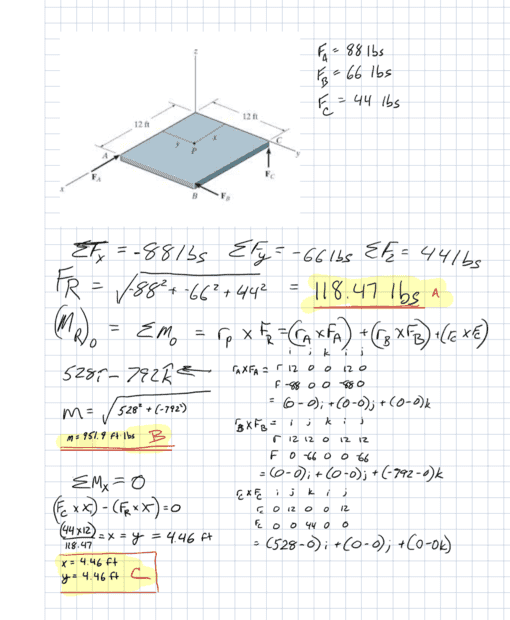# Finding the resultant force, moment, and x-y coordinates

j19063dc
Homework Statement:
I am trying to find help with finding the x any y coords of the resultant force, I have found the resultant force and moment but i think i may have run into problems finding the x and y coordinates. I know tha m_x' = sum Mx' but im unsure of the equation
Relevant Equations:
M_x' = sum Mx', My'=sumMy'